# Greek approach to measurement

#### N. J. Wildberger

Euclid does not deal directly with distance. The ancient Greeks understood the meaning of saying two segments in the same direction were in the ratio three to two', but they did not have a direct notion of distance, because that would have entailed an understanding of real numbers, which they did not have. Similarly, Euclid does not measure angle. To him, an angle was just the geometrical configuration consisting of two intersecting lines. In fact the modern notion of radian measure' is only a little more than a hundred years old.

Instead of distance and angle, the ancient Greeks believed that area is the fundamental quantity in planar geometry. To measure the separation of two lines, they measured the area of a square built on that line segment, a process called quadrature. A new theory of trigonometry, called rational trigonometry, was developed in 2005 by N. J. Wildberger (UNSW) in Divine Proportions: Rational Trigonometry to Universal Geometry, Wild Egg Books, Sydney, 2005, https://wildegg.com. Downloads from the book, supporting articles and links to reviews are available at https://wildegg.com/authors/. This note briefly motivates this new approach and lists the main laws, which require no transcendental circular functions.

# Pythagoras' theorem

There are two really important theorems about areas formed by three points. One is well known, the other much less so. Proposition 47 of Book 1 of Euclid's Elements states that for a right angled triangle, the square on the hypotenuse is the sum of the squares on the other two sides. This version of Pythagoras' theorem uses area, not distance. Area is an affine concept, in the sense that proportions between areas are invariant under affine transformations, which include linear transformations. To measure a line segment, Euclid measures the area of a square on that segment, a number which we call quadrance, being close to the word quadrature. Ifand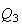are the three quadrances of a right trianglefor whichis perpendicular tothen Pythagoras' theorem can be stated asFor a triangle with rational points (vertices), the quadrances are rational numbers which can be determined combinatorially---by counting cells in a suitable grid lattice, as in the figure withand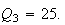Pythagoras' theorem has a sister theorem that Euclid does not mention, but which is implicit in work of Archimedes, and fundamental for rational trigonometry. It concerns the case when the three pointsand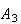are collinear. In this case the three quadrances satisfy the Triple quad formulaThe figure shows a case whereandAlthough algebraically more complicated, the Triple quad formula is in some sense a one-dimensional result, and easier to prove. Although Euclid did not have this theorem, one could argue that Archimedes did, as it is closely related to Herons' formula, known to Archimedes.

# Main laws of rational trigonometry

An angle is a circular distance, that is distance measured along a circular arc, and this is too complicated a concept to qualify as fundamental for measuring the separation of two lines. To define angles properly you require calculus, a logical point that is rarely acknowledged by educators. Teachers of trigonometry constantly rely on 90 - 45 - 45 and 90 - 60 - 30 triangles for examples and test questions. Once you get the hang of rational trigonometry and the much wider scope for explicit triangles that can be completely analysed, you will appreciate just how limiting classical trigonometry is. See Divine Proportions: Rational Trigonometry to Universal Geometry for a complete development of this new theory.

The true separation between lines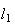andis captured by the concept of spread, which may be defined as the ratio of two quadrances. Supposeandintersect at the point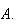Choose a point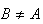on one of the lines, sayand letbe the foot of the perpendicular fromto.Then the spread s between l1 and l2 is

 s = s(l1, l2) = Q(B, C) / Q(A, B) = Q / R.

is ratio is clearly independent of the choice ofby Thales' theorem. The spread is defined between lines, not rays. Parallel lines are defined to have spread s = 0 while perpendicular lines have spread s = 1. you may check that the spread corresponding to 30° or 150° is s = 1/4. while the spread corresponding to 60° or 120° is s = 3/4.

When lines are expressed in Cartesian form, the spread becomes a rational expression in the coefficients of the lines. It therefore makes sense over arbitrary fields, although there is the possibility of null lines for which the denominator involved in the spread is zero. Note that in the triangleabove, the spread at the vertexand the spread at the vertex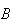sum to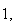on account of Pythagoras' theorem.

In diagrams a spread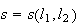is displayed beside a small line segment joining the two lines to distinguish it from angle. A spread protractor was created by Michael Ossmann and is available online at https://www.ossmann.com/protractor/.

So now a trianglehas quadrancesandas well as spreadsandas in the following diagram.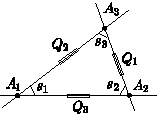The other main laws of rational trigonometry areThis is the Spread law, the analog of the Sine law. The analog of the Cosine law is the Cross law:The relationship between the three spreads of the triangle is the Triple spread law: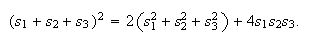The Triple quad formula, Pythagoras' theorem, the Spread law, the Cross law and the Triple spread formula are the five main laws of rational trigonometry. They are implicitly contained in the geometrical work of the ancient Greeks. As demonstrated at some length in `Divine Proportions', these formulas and a few additional secondary ones suffice to solve the majority of trigonometric problems, usually more simply, more accurately and more elegantly than the classical theory involving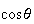,and their inverse functions. The same formulas extend to geometry over general fields and with arbitrary quadratic forms. See supporting articles at https://wildegg.com/authors.htm.

Example

The triangle with vertices,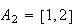andhas quadrances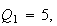andYou can check that the spreads are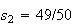and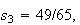and verify directly that all the laws are satisfied.

Since the laws are purely algebraic, they hold over a general field.

Example

If we work over the field with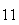elements, the above triangle has quadrancesandand spreads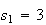,andThe above laws still hold.There is a collection of videos at YouTube that explains in detail the novel view point:

1. WildTrig1: Why trig is hard
2. WildTrig2: Quadrance via Pythagoras and Archimedes
3. WildTrig3: Spread, angles and astronomy
4. WildTrig4: Five main laws of rational trigonometry
5. WildTrig5: Applications of rational trigonometry
6. WildTrig6: Heron's formula viewed rationally
7. WildTrig7: Solving triangles with rational trigonometry
8. WildTrig8: Centers of triangles with rational trigonometry